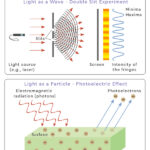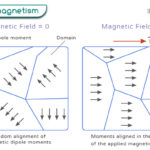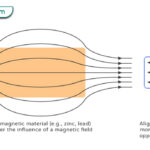Home / Physics / Kinetic Energy

# Kinetic Energy

## What is Kinetic Energy

The energy acquired by an object due to its motion is known as kinetic energy. The motion can be translational, rotational, vibrational, or a combination of all three.

According to Newton’s First Law, an object at rest will stay at rest unless acted by force. When force is applied, it does work on the object. Work means the transfer of energy from one form to another. In this case, the form into which energy transforms is kinetic energy. The object attains kinetic energy and moves with a specific velocity.

## Kinetic Energy Equation

### How to Find Kinetic Energy

Kinetic energy is a scalar quantity, not a vector. It takes positive values that depend on two factors – velocity and mass. It cannot be negative since mass cannot be negative. The formula for kinetic energy is given by,

K.E. = ½ mv2

Where,

K.E.: kinetic energy

m: mass

v: velocity

SI Unit: Joule or J (1 J = 1 kg m2/s2)

Cgs Unit: Erg (107 erg = 1 J)

Dimensions: [ML2T-2]

For the above equation, it is clear that increasing velocity increases kinetic energy. Similarly, an object with a higher mass will have higher kinetic energy.

### Change in Kinetic Energy

An object can start from rest and acquire velocity. An object can also change its velocity during its motion. Suppose the object moves with a velocity vi, the initial velocity. A force alters its motion such that its velocity changes to vf, which is the final velocity. Then, the change in kinetic energy is,

ΔK.E. = ½ mvf2 – ½ mvi2

Now, work is being done on the object to change its motion. The change of kinetic energy is equal to the work done W. Therefore,

W = ΔK.E.

This equation is known as the work-energy theorem.

### Rotational Kinetic Energy

An object rotating about its axis also has kinetic energy. Its kinetic energy depends on angular velocity and moment of inertia. For rotational motion, the expression for kinetic energy is different from linear motion. The formula is,

K.E. = ½ Iω2

Where,

I: moment of inertia

ω: angular velocity

An example of rotational kinetic energy is a merry-go-round.

Suppose an object executes both translational and rotational motion. In that case, the total kinetic energy is the sum of kinetic energy due to each motion.

K.E.Total = ½ mv2 + ½ Iω2

### Vibrational Kinetic Energy

A vibrating object has kinetic energy. For example, a spring oscillates with kinetic energy given by,

K.E. = ½ kx2

Where,

k: spring constant

## Kinetic Energy Examples

Any object that has mass is capable of moving has kinetic energy. Here are a few examples.

• A bird flying in the air
• A ship sailing in the sea
• A car driving on the road
• A rocket launching into space
• Water flowing down a stream
• Planets orbiting around the sun with a gravitational force
• A windmill with its blades rotating
• A bullet fired from a gun
• An oscillating pendulum
• An archer releasing an arrow from a bow
• A roller coaster in descent

The following image shows a few examples of kinetic energy.

## Types of Kinetic Energy

There are five different forms of kinetic energy.

1. Mechanical Energy: It is the energy associated with the mechanical movement of objects.

Examples:

• A bowling ball rolling down an alley
• A soccer ball moving through the air

2. Electrical Energy: Also known as electricity, it is caused by electron flow along a conductor.

Examples:

• A table lamp turned on
• Electrolysis

3. Sound Energy: It is caused by the motion of vibrating air particles. When the vibrations reach the ears, the brain perceives them as sound.

Examples:

• Human voice
• Sound of drums

4. Radiant Energy: It is caused by oscillating electric and magnetic fields, resulting in electromagnetic waves. Particles or waves carry the energy.

Examples:

• Light emitted by incandescent light bulbs

5. Thermal Energy: Also known as heat energy, it is caused by atoms and molecules moving and colliding. The faster the atoms move, the hotter the object will be.

Examples:

• Boiling water in a pan
• Baking food in an oven

## Summary

The following points summarize all that we have learned about kinetic energy.

• Associated with a moving object
• Increases or decreases with the change in velocity
• Greater for heavier mass
• Can transform into other types of energy
• Measured in the unit of Joules

## Solved Problems

P.1. A ball weighs around 2 kg and travels at 15 meters per second. What is its kinetic energy?

Soln.: Given,

m = 2 kg

v = 15 m/s

Therefore,

K.E. = ½ (2 kg) (15 m/s)2

Or, K.E. = 225 J

P.2. Calculate the kinetic energy of a 78 kg person walking with a speed of 1.2 m/s?

Soln.: Given,

m = 78 kg

v = 1.2 m/s

K.E. = ½ mv2

Or, K.E. = ½ (78 kg) (1.2 m/s)2

Or, K.E. = 56.2 J

P.3. Calculate the mass of an object moving at 25 m/s with a kinetic energy of 1500 J.

Soln.: Given,

K.E. = 1500 J

v = 25 m/s

The kinetic energy is,

K.E. = ½ mv2

Or, m = 2 K.E./v2

Or, m = 2 x 1500 kg m2s-2 /(25 ms)2

Or, m = 4.8 kg

P.4. Work done by a force on a moving object is 150 J. The object was traveling at a speed of 2.5 m/s. Find the new speed of the object if the mass of the object is 1.5 kg.

Soln.: Given,

W = 150 J

vi = 2.5 m/s

m = 1.5 kg

Therefore,

W = ΔK.E.

Or, W = ½ m (vf2 – vi2)

Or, vf2 = 2W/m + vi2

Or, vf2 = 2 x 150 kg ms-1 /1.5 kg + (2.5 ms-1)2 = 206 m2/ss

Or, vf = √206 m2/ss = 14.3 m/s

## FAQs

Q.1. Is kinetic energy stored energy?

Ans. Kinetic energy is not a stored energy. Rather, it is a release energy.

Q.2. What material can absorb kinetic energy?

Ans. An example of an object that can absorb kinetic energy is aluminum.

Q.3. What is a source of kinetic energy?

Ans. A source of kinetic energy is wind.

Article was last reviewed on Monday, February 7, 2022

### Related articlesWave-Particle DualityPhotoelectric EffectFerromagnetismDiamagnetism